Superconductivity

Start this free course now. Just create an account and sign in. Enrol and complete the course for a free statement of participation or digital badge if available.

Free course

# 3.5 The screening current

The London equations relate the magnetic field in a superconductor to the superconducting current density, and we derived the dependence of field on position by eliminating the current density. However, if we eliminate the magnetic field instead, we can derive the following equation for the current density: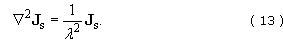## Exercise 4

Derive Equation 13 by taking the curl of both sides of Equation 9 and then using Ampère's law to eliminate curl B. Assume that the currents are steady.

Taking the curl of both sides of Equation 9 and using Ampère's law, curl B = μ﻿0﻿J﻿s, to eliminate B, we find that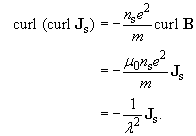We now use a standard vector identity to rewrite the curl(curl Js) term: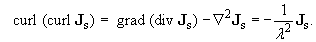For our steady-state situation, where ∂﻿ρs/∂﻿t = 0, the equation of continuity, ∂﻿ρs/∂t + div Js = 0, reduces to div J﻿s = 0, so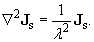Equation 13 has exactly the same form as Equation 11. So for the planar symmetry that we discussed earlier – superconducting material occupying the region z > 0 (Figure 14) – the solution for the current density will have the same form as Equation 12, that is,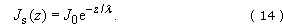This equation gives no indication of the absolute magnitude or direction of current flow, but we can deduce this by using Ampère's law, curl B = μ﻿0﻿J﻿s. In the planar situation that we are considering, B = Bx (zex, so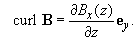Then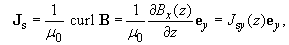where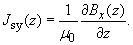But we know that Bx(z) = B﻿0﻿e﻿−﻿z﻿/﻿λ (Equation 12), so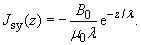Thus the current that screens the interior of the superconductor from an applied field flows within a thin surface layer, which has a thickness characterised by the penetration depth λ, and the current flows parallel to the surface and in a direction perpendicular to the magnetic field, as shown in Figure 17.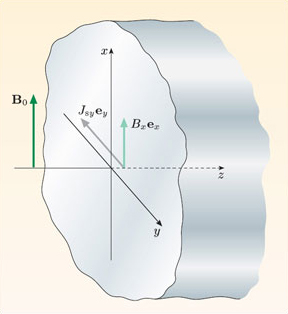Figure 17 The magnetic field and current density vectors in the surface layer of a superconductor.

## Exercise 5

The number density of free electrons in tin is 1.5 × 10﻿29 m﻿−﻿3. Calculate the penetration depth predicted by the London model, assuming that all of the free electrons are superconducting, and compare the result with the value measured by Schawlow and Devlin.

From Equation 10, the penetration depth is given by λ = (m/μ0nse2)1/2, so (in metres)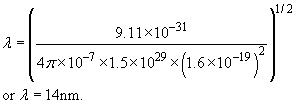This value, predicted by the London model, is about a quarter of the measured value.

The numerical discrepancy between the London model prediction for the penetration depth of tin and the experimentally measured value indicates that this model has limitations. One limitation is that the model is essentially a local model, relating current density and magnetic field at each point. Superconductivity, though, is a non-local phenomenon, involving coherent behaviour of the superconducting electrons that are condensed into a macroscopic quantum state. The characteristic distance over which the behaviour of the superconducting electrons is linked is known as the coherence length, ξ, introduced in Section 1. This distance represents the distance over which the number density of the superconducting electrons changes, and is a measure of the intrinsic non-local nature of the superconducting state. The London local model is a good description if ξ ≪ λ, that is, the coherence length is much shorter than the distance λ over which the fields and current density are changing. Since the penetration depth increases sharply as the temperature approaches the critical temperature (Figure 16), the London model becomes a good approximation in this limit. More importantly, the coherence length of superconductors decreases as the critical temperature increases and as the scattering time for normal electrons decreases. Both of these effects mean that the coherence length is short compared with the penetration depth in alloy and ceramic superconductors, so the London local model is a good approximation in these cases too, and predicted and experimental results for the penetration depth are in good agreement.

For pure elements, well below their critical temperatures, the penetration depth is generally much shorter than the coherence length, so a local model is not appropriate. In this limit, the number density of superconducting electrons does not reach the bulk value until a distance of the order of ξ, which is greater than λ, from the surface, and the reduced value of n﻿s accounts for the discrepancy between the predicted and experimental results for the penetration depth of tin discussed earlier.

The ratio of the penetration depth to the coherence length is an important parameter for a superconductor, and we shall return to this subject in Subsection 4.2.

SMT359_1